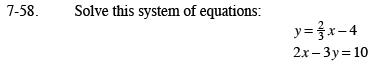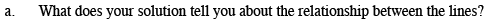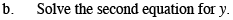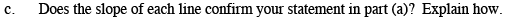Home > CAAC > Chapter 7 > Lesson 7.2.1 > Problem7-58

7-58.
1. Solve this system of equations: Homework Help ✎

y =− 4

2x − 3y = 10

1. What does your solution tell you about the relationship between the lines?

2. Solve the second equation for y.

3. Does the slope of each line confirm your statement in part (a)? Explain how.Since the first equation is in y = form, solve using substitution.

$2x \minus 3y = 10$

$2x-3\left(\frac{2}{3}x-4\right)=10$

2x − 2x + 12 = 10

12 = 10 What does this mean?What does it mean when you solve a system of equations with two lines and there is no solution?

The lines do not intersect.Subtract 2x from both sides.

Divide by −3.The lines have the same slope. What kind of lines have the same slope?